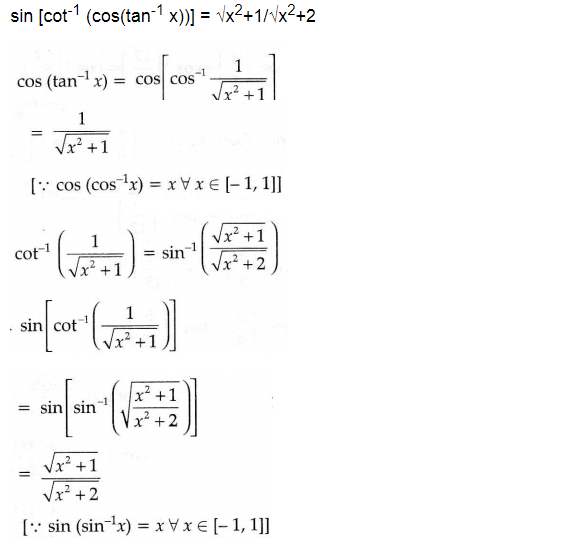# sin[cot-1{cos(tan-1x)}]

10 years ago

sin (cot-1 {cos (tan -1x)})

tan-1 x = A  => tan A =x

sec A = √(1+x2) ==>  cos A = 1/√(1+x2)    so   A =  cos-1(1/√(1+x2))

sin (cot-1 {cos (tan -1x)}) = sin (cot-1 {cos (cos-1(1/√(1+x2))})

=sin (cot-1 {(1/√(1+x2))})

if cot-1 {(1/√(1+x2))} = B

{(1/√(1+x2))} = cotB  ==>  cosec B = {(√[(2+x2)/(1+x2)])}

sin B = {(√[(1+x2)/(2+x2)]} ==>  B  = sin -1 ({(√[(1+x2)/(2+x2)]})

sin {sin -1 ({(√[(1+x2)/(2+x2)]})} = √[(1+x2)/(2+x2)]

10 years agoKushagra Madhukar
3 years ago
Hello student

Let, sin (cot-1 {cos (tan -1x)}) = T

Let, tan-1 x = A                                   or                    T = sin (cot-1 {cos (A)})

=> tan A = x;  sec A = √(1+x2) ==>  cos A = 1/√(1+x2)

T = sin (cot-1 {cos (A)})  =   sin (cot-1 {(1/√(1+x2))})

Let, cot-1 {(1/√(1+x2))} = B                  or                  T = sin (B)

{(1/√(1+x2))} = cotB  ==>  cosec B = {(√[(2+x2)/(1+x2)])} ==> sin B = {(√[(1+x2)/(2+x2)]}

Hence, T = sin (B) = √[(1+x2)/(2+x2)]

Therefore, the answer is, T = √[(1+x2)/(2+x2)]Yash Chourasiya
3 years ago
Dear Student

Please see the solution in the attachment.